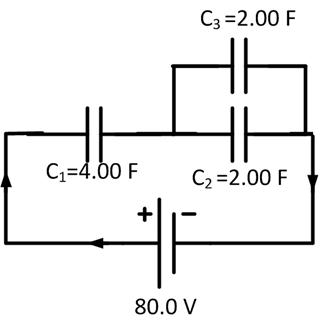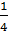### Sample ProblemWhat is the total charge of the circuit above? C

#### Solution

First, we need calculate the equivalent capacitance of the circuit.

C2 + 3 = 2.00 F + 2.00 F = 4.00 F=+=+== 2.00 F

Q = CV = 2.00 F × 80.0 V = 160 C